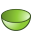# Paraboloid

Create a paraboloid.

On the Construct tab, in the Create Surface group, click theParaboloid icon.
Tip: Press S,4 to use the shortcut key.Centre point (C) The apex of the paraboloid. Radius (R) The radius of the paraboloid aperture, parallel to the UV plane. Focal depth (F) The focal depth (F) of the paraboloid is the distance from the centre point (C) to the focal point. If this is negative, the paraboloid is oriented towards the negative N axis.

The focal depth is related to the dimensions of paraboloid by

(1) $f=\frac{{R}^{2}}{4h}$
where (H) denotes the distance from the centre point (C) to the aperture centre of the paraboloid.